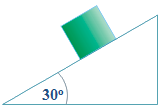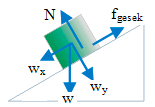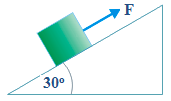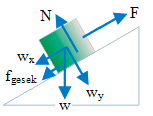# Motion on the rough inclined plane with the friction force – application of Newton’s law of motion problems and solutions

1. Object’s mass = 2 kg, acceleration due to gravity = 9.8 m/s2, coefficient of the static friction = 0.2, coefficient of the kinetic friction = 0.1. Is the object at rest or accelerating? If the object is accelerated, find (a) the net force (b) magnitude and direction of the box’s acceleration!SolutionKnown :

Mass (m) = 2 kg

Acceleration due to gravity (g) = 9.8 m/s2

Coefficient of the static friction (μs) = 0.2

Coefficient of the kinetic friction (μk) = 0.1

Weight (w) = m g = (2)(9.8) = 19.6 Newton

The horizontal component of the weight (wx) = w sin 30o = (19.6)(0.5) = 9.8 Newton

The vertical component of th weight (wy) = w cos 30o = (19.6)(0.5√3) = 9.8√3 Newton

The normal force (N) = wy = 9.8√3 Newton

Force of the static friction (fs) = (0.2)(9.8√3) = 1.96√3 Newton = 3.39 Newton

Force of the kinetic friction (fk) = (0.1)(9.8√3) = 0.98√3 Newton = 1.69 Newton

Solution :

Object is at rest if wx < fs, object is moving down if wx > fs.

wx = 9.8 Newton and fs = 3.39 Newton.

(a) the net force

F = wx – fk = 9.8 – 1.69 = 8.11 Newton

(b) magnitude and direction of the acceleration

F = m a

8.11 = (2) a

a = 4.05

Magnitude of the acceleration = 4.05 m/s2 and direction of the acceleration = downward.

[irp]

2. Object’s mass = 4 kg, acceleration due to gravity = 9,8 m/s2. Coefficient of the kinetic friction = 0.2 and coefficient of the static friction = 0.4. Magnitude of the force F = 40 Newton. The object is at rest or slides down ? If the object slides down, find (a) the net force (b) magnitude and direction of the acceleration!SolutionKnown :

Mass (m) = 4 kg

Acceleration due to gravity (g) = 9.8 m/s2

The coefficient of the static friction (μs) = 0.4

The coefficient of the kinetic friction (μk) = 0.2

Weight (w) = m g = (4)(9.8) = 39.2 Newton

The horizontal component of the weight (wx) = w sin 30o = (39.2)(0.5) = 19.6 Newton

The vertical component of the weight (wy) = w cos 30o = (392)(0..5√3) = 19.6√3 Newton

The normal force (N) = wy = 19.6√3 Newton = 33.95 Newton

the static friction force (fs) = μs N = (0,4)(33.95) = 13.58 Newton

The kinetic friction force (fk) = μk N = (0.2)(33.95) = 6.79 Newton

F = 40 Newton

Solution :

The object slides down if F < wx + fs. The object slides up if F > wx + fs.

F = 40 Newton, wx = 19.6 Newton and fs = 13.58 Newton.

F is greater than wx + fs so the object slides up.

(a) The net force

F = F – wxfk = 40 – 19.6 – 6.79 = 13.61 Newton

(b) The magnitude and direction of the acceleration

F = m a

6.4 = (4) a

a = 1.6

The magnitude of the acceleration is 1.6 m/s2 and direction of the acceleration is upward.

[irp]

[wpdm_package id=’481′]